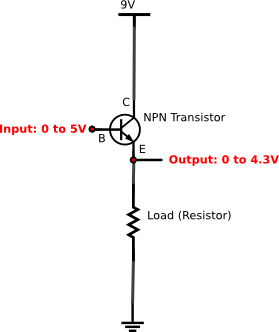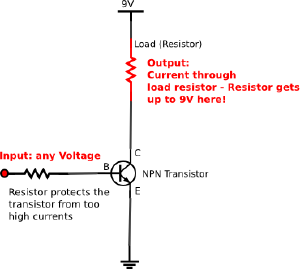Most widely used: npn transistors. (also called bipolar transistor or just junction transistor. Note: bipolar doesn't mean they are usable for AC currents! - it just tells us about the internal arrangement of semi conductors.)

Bipolar transistors have 3 terminals - Emitter, Base and Collector. In most circuits we are using the base as "input" - the Emitter and Collector act either like a variable resistor (or like a switch).

## Contents

### Amplifying: Emitter Follower

The emitter follower - also called the "Common_collector" is a circuit where the output voltage follows the input voltage (therefore the name). While the input current can be pretty low the current through a load can be much higher - which is one of the main applications of this circuit.

The emitter follower comes handy whenever you have a tiny voltage source that isn't capapble of delivering a desired current for an application and you want to amplify that. Using an extra voltage supply and a transistor you can do this. For example a potentiometer can deliver a variable voltage - however as soon as you use a current from that voltage divider the voltage will drop rapidly which is not intended. An emitter follower will elegantly solve this problem in many cases. Have a look at the picture: Connect the output of the voltage divider (or generally the weak voltage source) to the base of the transistor. Then then the load you want to drive (for example a motor) is put between ground and the Emitter. The supply voltage is connected to the collector of the transistor. If you now adjust the potentiometer to deliver 0V the motor is off. If you turn it all the way up - giving us for example 5V the motor will also get approximately 5V. At the center the voltage divider gives us 2.5V - the motor will also have approximately 2.5V. In reality there's a little voltage drop depending on the transistor's material. Silicium transistors will take approx. 0.7V - so the maximum is only 4.3V. Also when your input voltage is below 0.7V, the output remains almost zero all the time. If your application doesn't allow this better us an operational amplifier (opamp).

An important property is the independance of the supply voltage for the load. If your input is ranging from 0 to 5 - the output will do the same - no matter how high the supply voltage is. The remaining voltage above 5V will just be wasted in the transistor and create heat - intended or not.

### Switching: common-emitter amplifier

Firstly - don't confuse it with the emitter follower because of the similarity of the name. This circuit is useful to switch loads on and off by a small control voltage. The name is derived of by its electrical connection. If you have 10 of these circuits all emitters are connected together - they have a common terminal for their emitters.

The common emitter amplifier is mainly used to switch a power hungry device on and off using only a weak control signal (hungre devices: high power LEDs, motors, ...) For example an Arduino digital pin is capable of a 40 mA current.

The circuit makes use of the amplification factor (the hfe measurement on your digital multimeter displays this factor). For example a transistor with an amplification factor of 100 is used. If we feed a current of 1mA into the base (more precisely: from the base to the emitter) the transistor will allow a current of 100mA flow from collector to the base. Imagine we are using a motor which usually draws 200mA - the current is limited by the transistor. The transistor appears like a series resistor to the motor, limiting the current to 100mA. Now imagine we do the same with 1.5 mA. The transistor will allow a current of 150mA through the motor. Apparently its resistance is now smaller than before. Now we give a current of 2mA into the base - et voila - the motor gets the desired current of 200mA. Now we increase the current to 3mA - what might happen? - Nothing. The motor was already directly connected to the supply voltage - taking its 200mA. As the transistor is only acting as a variable resistor the transistor can not force an even higher current through the motor. The operation above the needed current is also called "saturation". If we want to switch something we ususally use this - and to be sure it is definitely the lowest possible resistance we can get we let a larger current flow through the base than needed. Transistors are varying their amplification factor with temperature and also have a widely spread range of the amplification factor - even coming out of the same factory the same day. To be sure the transistor is not limiting the current by accident, just use an over sized base current - defined by the resistor attached to the base.

Example:

• Needed current through load is 1000mA
• Amplification factor is 100 (but we assume only 50)
• so the base current has to be at least 20mA

At a given voltage of 5V we can calculate the needed resistance: R = V / I = 5V / 0.02 A = 250 ohm.